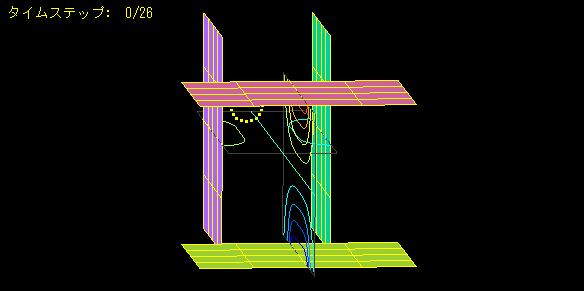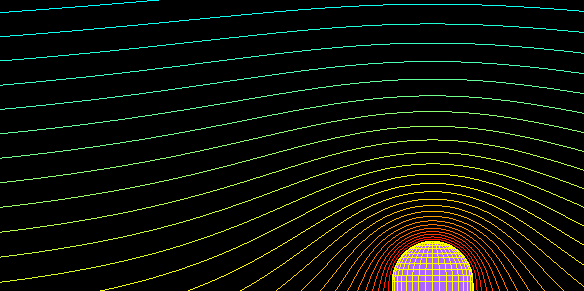Solver Trajectory Analysis ELF/BEAM ≫

The following calculation times are on a Core i7 6700K PC (in seconds).

#### Magnetic lens consisting of two air-core coils

Calculation time: < 1 secondThis is the electron trajectory when an electron beam is injected into a magnetic lens consisting of two air-core coils.
The trajectory is calculated by placing eight electrons on the circle.
They are collected at the center while rotating around the central axis.

#### Undulator light source

Calculation time: 2 secondsThis is an example of an undulator light source analysis.
Multiple magnets are aligned with alternating poles, and the electron trajectory is calculated to be meandering.
Radiation is produced at this time.
Even if the model is created with coarse elements, the magnetic field is calculated accurately until the vicinity of the magnet, so the electron trajectory can be calculated with high accuracy.

#### Trajectory analysis of electrons with two sets of parallel electrodes

Calculation time: < 1 secondTwo sets of parallel electrodes are placed orthogonally to analyze electron trajectories.
This is an example of applying voltage to parallel electrodes and performing electric field analysis in ELFIN to analyze the trajectory of electrons ejected in a circular pattern.

#### Trajectory analysis of electrons ejected from a needle electrode

Calculation time: 2 secondsElectron trajectory analysis.
This is an example of applying voltage to a needle electrode and a flat electrode for electron acceleration, performing electric field analysis with ELFIN, and simulating the trajectory of electrons ejected from the needle electrode.
The needle electrode can be changed to a dielectric, allowing finely curved surfaces to be modeled with high accuracy.It is possible to stop the electron trajectory when there are obstacles in the middle of the trajectory.
Obstacles (electrodes and walls) of arbitrary shapes can be created.
Secondary electrons can be emitted from the wall.

a:10429 t:2 y:0